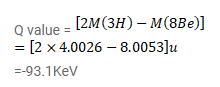# Calculate the Q-value of the fusion reaction

Question:

Calculate the Q-value of the fusion reaction

${ }^{4} \mathrm{He}+{ }^{4} \mathrm{He}={ }^{8} \mathrm{Be}$

Is such a fusion energetically favorable? Atomic mass of ${ }^{8} \mathrm{Be}$ is $8.0053 \mathrm{u}$ and that of $4 \mathrm{He}$ is $4.0026 \mathrm{u}$.

Solution: